## batgrbproduct Analysis for Trigger=617149

### Notes:

• 1) No attempt has been made to eliminate the non-significant decimal places.
• 2) This batgrbproduct analysis is done of the amount of data for this trigger downloaded to date.
• 3) This batgrbproduct analysis is using the flight position for the mask-weighting calculation.
```======================================================================
BAT GRB EVENT DATA PROCESSING REPORT
Process Script Ver: batgrbproduct v2.46
Process Start Time (local time): Sat Nov  1 03:39:32 EDT 2014
Host Name: bat1
======================================================================

Trigger: 00617149      Segment: 000

BAT Trigger Time: 436460217.792   [s; MET]
Trigger Stop: 436460225.984   [s; MET]
UTC: 2014-10-31T14:56:45.065320    [includes UTCF correction]

BAT
RA: 356.931700786733   Dec: 41.3655291034627  [deg; J2000]

Catalogged Source?: NO
Point Source?:      YES
GRB Indicated?:     YES    [ by BAT flight software ]
Image S/N Ratio:    7.44
Image Trigger?:     NO
Rate S/N Ratio:     8.94427190999916  [ if not an image trigger ]
Image S/N Ratio:    7.44

Flight Position:   [ source = BAT ]
RA: 356.931700786733   Dec: 41.3655291034627  [deg; J2000]

Ground Position:   [ source = BAT pre-slew burst ]
RA: 356.882859439076   Dec: 41.355294913241  [deg; J2000]
{ 23h 47m 31.9s , +41d 21' 19.1" }
+/- 2.21942263432925 [arcmin] (estimated 90% radius based on SNR)
+/- 0.898987403006968 [arcmin] (formal 1-sigma fit error)
SNR: 9.73844897620899

Angular difference between Flight and Ground position is 2.283630 arcmin

Partial Coding Fraction: 1.015625    [ including projection effects ]

Duration
T90: 16 +/- 3.60555127546399
Measured from: 1.20800012350082
to: 17.2080001235008     [s; relative to TRIGTIME]
T50: 9 +/- 4.47213595499958
Measured from: 4.20800012350082
to: 13.2080001235008     [s; relative to TRIGTIME]

Fluence
Peak Flux  (peak 1 second)
Measured from: 4.20800000429153
to: 5.20800000429153     [s; relative to TRIGTIME]
Total Fluence
Measured from: 1.20800000429153
to: 18.2080000042915     [s; relative to TRIGTIME]

Band 1     Band 2     Band 3     Band 4
15-25      25-50     50-100    100-350     keV
Total     0.116985   0.113627   0.112450   0.060711
0.022560   0.023246   0.020339   0.017514 [error]
Peak      0.017074   0.011853   0.007756   0.013905
0.005493   0.005692   0.004992   0.004109 [error]

[ fluence units of on-axis counts / fully illuminated detector ]

======================================================================

=== Fluence/Peak Flux Summary ===

Model    Band1    Band2    Band3    Band4    Band5    Total
15-25    25-50   50-100  100-350   15-150   15-350   keV
Fluence   Power-law 2.85e-08 5.71e-08 8.91e-08 3.07e-07 2.48e-07 4.81e-07   erg/cm2
Error 9.42e-09 1.05e-08 2.08e-08 1.72e-07 4.99e-08 1.84e-07   erg/cm2
Peak flux Power-law 1.20e-01 1.12e-01 7.24e-02 7.22e-02 3.34e-01 3.77e-01   ph/cm2/s
Error 1.00e-01 4.36e-02 5.75e-02 1.06e-01 1.26e-01 1.51e-01   ph/cm2/s

Fluence   Cutoff-PL 2.92e-08 5.75e-08 8.78e-08 2.90e-07 2.46e-07 4.64e-07   erg/cm2
Error 9.40e-09 1.05e-08 2.06e-08 1.83e-07 4.77e-08 1.87e-07   erg/cm2
Peak flux Cutoff-PL 1.23e-01 1.12e-01 7.07e-02 6.76e-02 3.34e-01 3.73e-01   ph/cm2/s
Error 7.51e-02 4.24e-02 5.75e-02 1.12e-01 1.26e-01 1.47e-01   ph/cm2/s

Fluence        Band -------- -------- -------- -------- -------- --------   erg/cm2
Error -------- -------- -------- -------- -------- --------   erg/cm2
Peak flux      Band -------- -------- -------- -------- -------- --------   ph/cm2/s
Error -------- -------- -------- -------- -------- --------   ph/cm2/s
```
```
=== Time-averaged spectral analysis ===

Model Ph_index Ph_90err Epeak  Epeak_90err Norm     Nomr_90err chi2   dof
PL    1.357    0.352    -      -           1.50e-03 2.57e-04   56.280 57
CPL   2.461    0.000     153.3    0.0      4.16e-04 0.00e+00   52.760 56

=== 1-s peak spectral analysis ===

Model Ph_index Ph_90err Epeak  Epeak_90err Norm     Nomr_90err chi2   dof
PL    1.628    0.885    -      -           2.58e-03 1.18e-03   56.270 57
CPL   3.971    0.000     459.6    0.0      2.00e-04 0.00e+00   55.010 56

Model      : spectral model: simple power-law (PL) and cutoff power-law (CPL)
Ph_index   : photon index: E^-{Ph_index} (minus sign is not included in the index)
Ph_90err   : 90% error of the photon index
Epeak      : Epeak in keV
Epeak_90err: 90% error of Epeak
Norm       : normalization at 50 keV in PL model and at 1 keV in CPL model
Nomr_90err : 90% error of the normalization
chi2       : Chi-Squared
dof        : degree of freedom
```
```
=== T90/T50 calculation in the 50-300 keV band (BATSE band) using 64 ms lc ===

T90 in the 50-300 keV band: 7.936000 sec.
T50 in the 50-300 keV band: 4.096000 sec.
```
```
=== Table of the duration information ==

Value   tstart    tstop
T100   17.000    1.208   18.208
T90    16.000    1.208   17.208
T50     9.000    4.208   13.208
Peak    1.000    4.208    5.208
```

### Image

#### Pre-slew 15-350 keV image (Event data; bkg subtracted)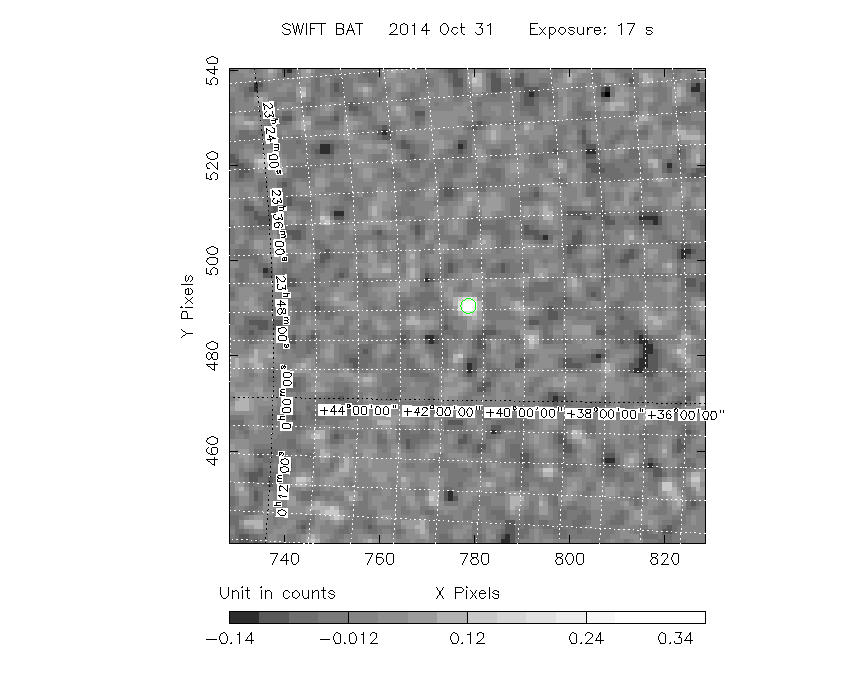```#   RAcent  DECcent POSerr  Theta    Phi     Peak Cts     SNR  Name
356.8829 +41.3553 0.0198   12.1   -7.8       0.4043     9.7  TRIG_00617149
------------------------------------------

Foreground time interval of the image:
1.208  18.208 (delta_t =  17.000 [sec])
Background time interval of the image:
-239.792   1.208 (delta_t = 241.000 [sec])
```

### Notes:

• 1) All plots contain as much data as has been downloaded to date.
• 2) The mask-weighted light curves are using the flight position.
• 3) Multiple plots of different time binning/intervals are shown to cover all scenarios of short/long GRBs, rate/image triggers, and real/false positives.
• 4) For all the mask-weighted lightcurves, the y-axis units are counts/sec/det where a det is 0.4 x 0.4 = 0.16 sq cm.
• 5) The verticle lines correspond to following: green dotted lines are T50 interval, black dotted lines are T90 interval, blue solid line(s) is a spacecraft slew start time, and orange solid line(s) is a spacecraft slew end time.
• 6) Time of each bin is in the middle of the bin.

#### 1 s binning

##### From T0-310 s to T0+310 s##### From T100_start - 20 s to T100_end + 30 s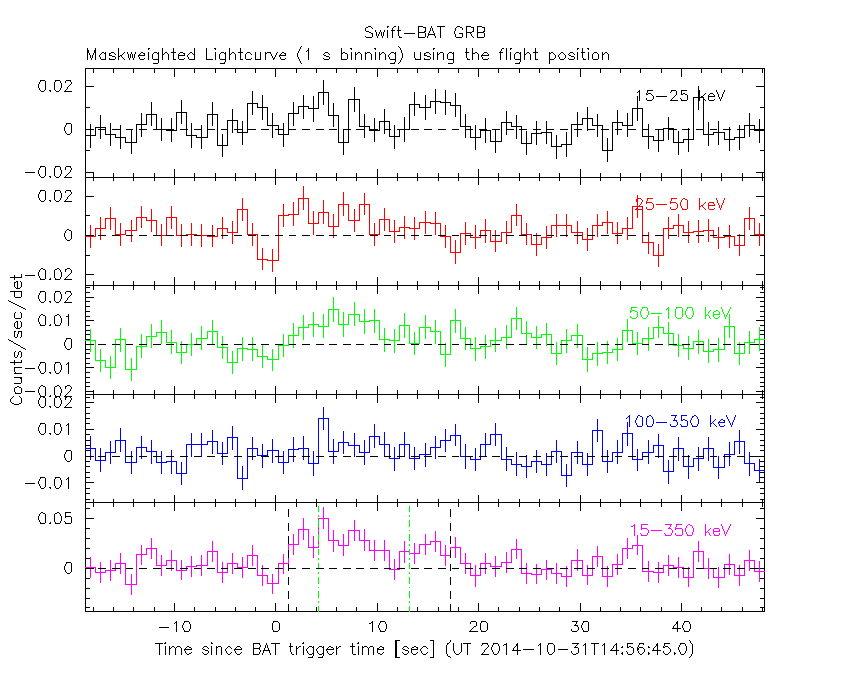##### Full time interval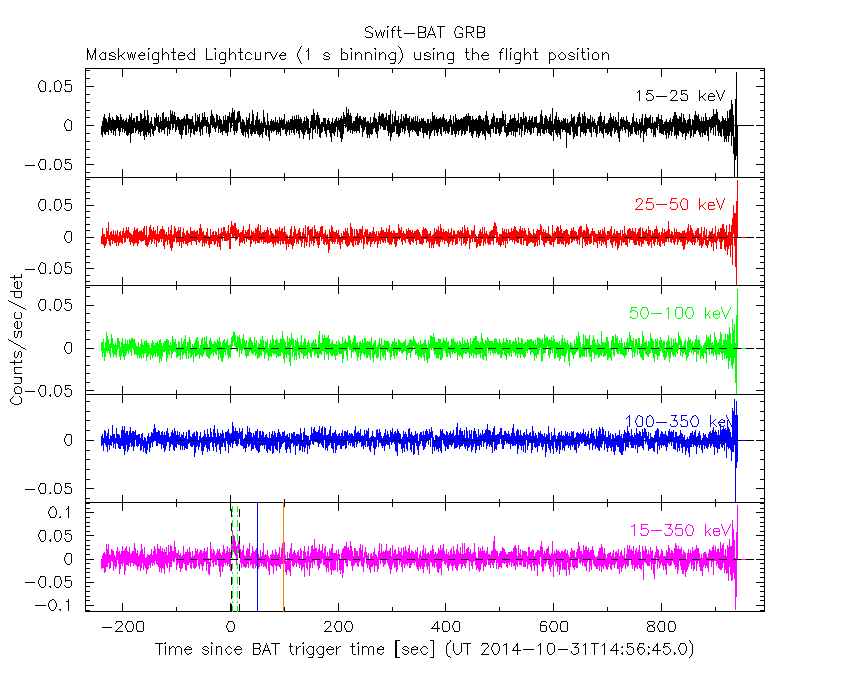#### 64 ms binning

##### Full time interval##### From T100_start to T100_end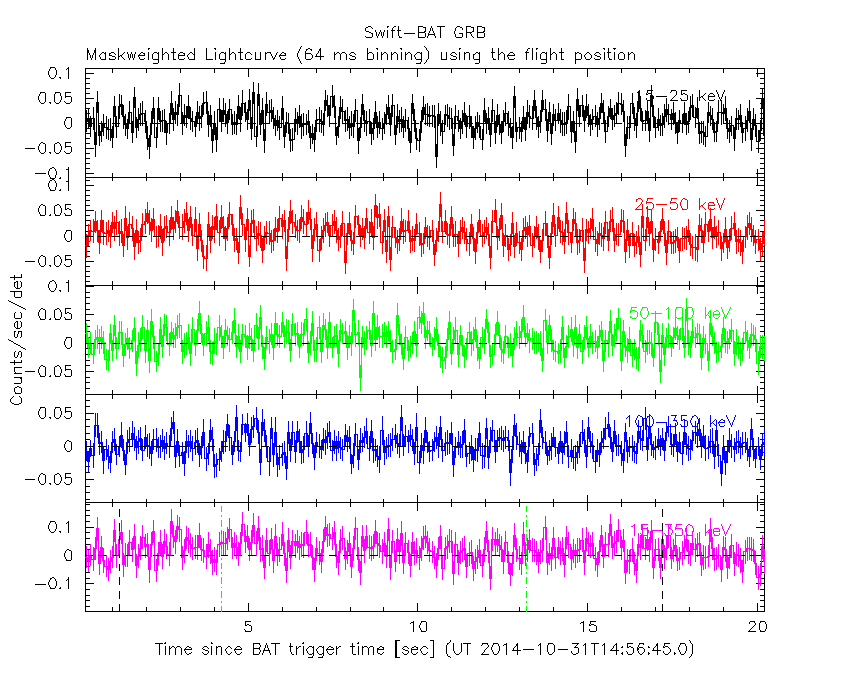##### From T0-5 sec to T0+5 sec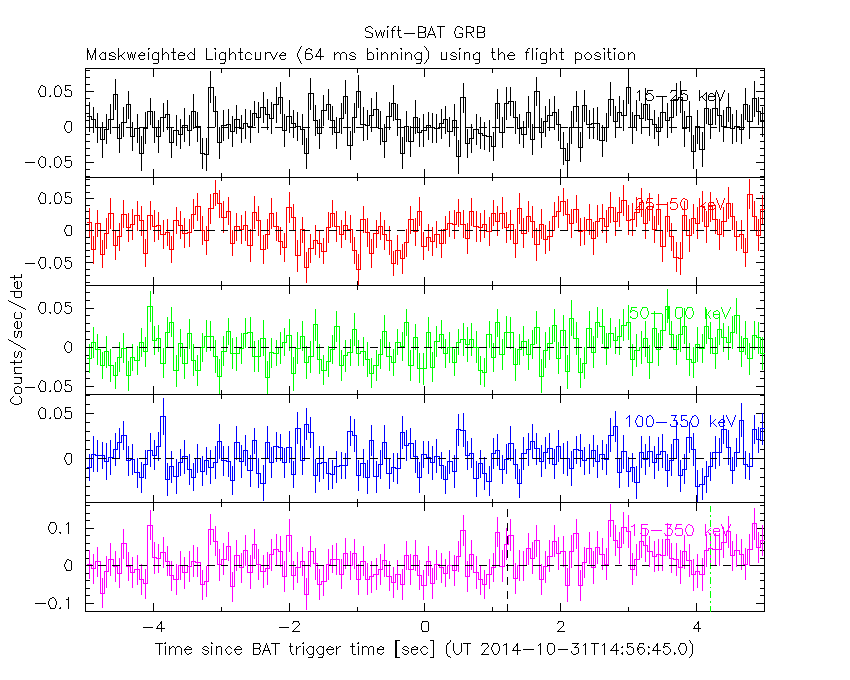#### 16 ms binning

##### From T100_start-5 s to T100_start+5 s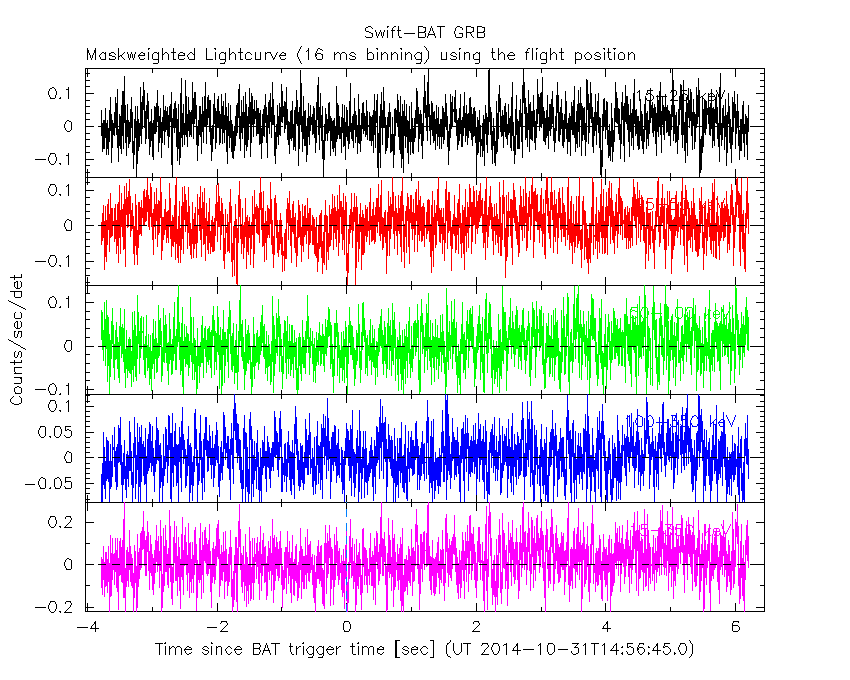#### 8 ms binning

##### From T100_start-3 s to T100_start+3 s#### 2 ms binning

##### From T100_start-1 s to T100_start+1 s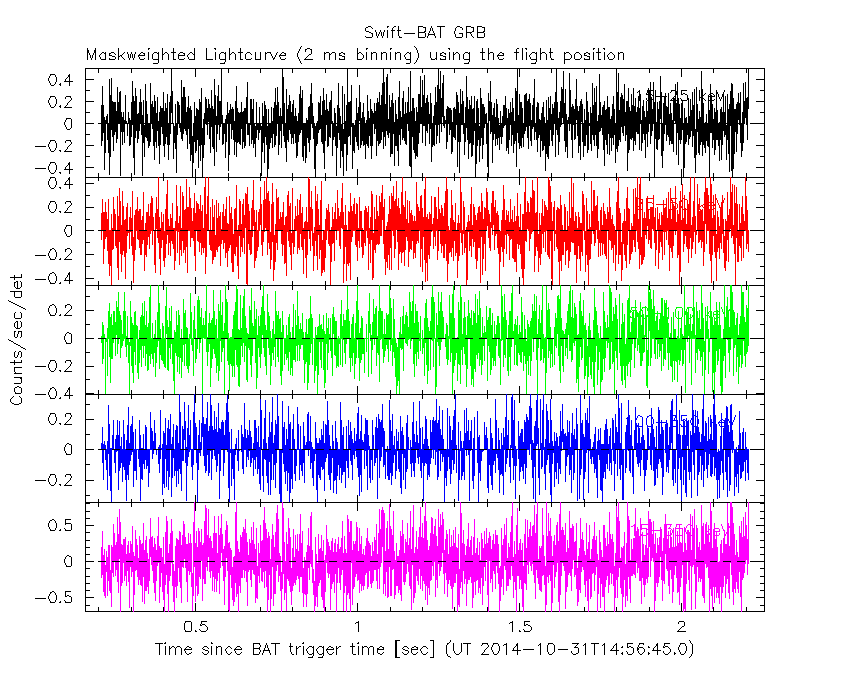#### SN=5 or 10 sec. binning (whichever binning is satisfied first)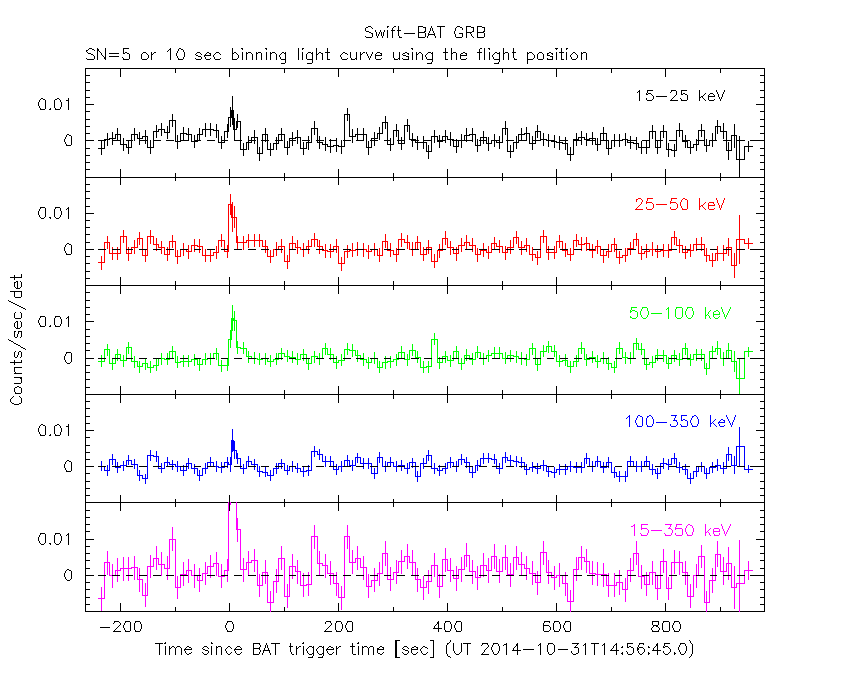#### SN=5 or 10 sec. binning (T < 200 sec)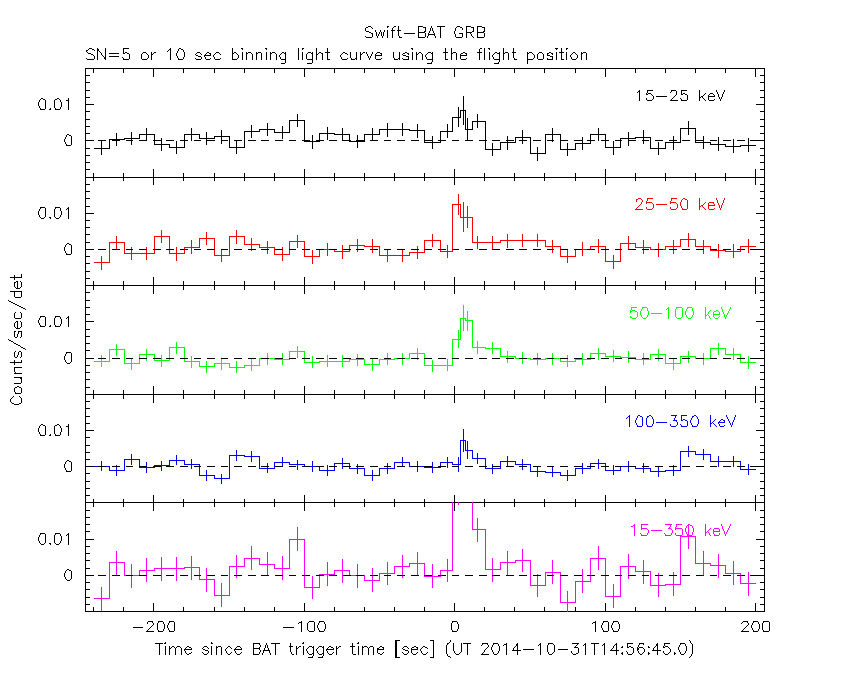### Notes:

• 1) The fitting includes the systematic errors.
• 2) For long bursts, a spectral fit of the pre-slew DRM will introduce some errors in both a spectral shape and a normalization if the PHA file contains a lot of the slew/post-slew time interval.
• 3) For fits to more complicated models (e.g. a power-law over a cutoff power-law), the BAT team has decided to require a chi-square improvement of more than 6 for each extra dof.
• 4) For short bursts (T90<1sec), the specrtum is also fit with Blackbody, OTTB, and Double Blackbody.

#### Time averaged spectrum fit using the pre-slew DRM

##### Power-law model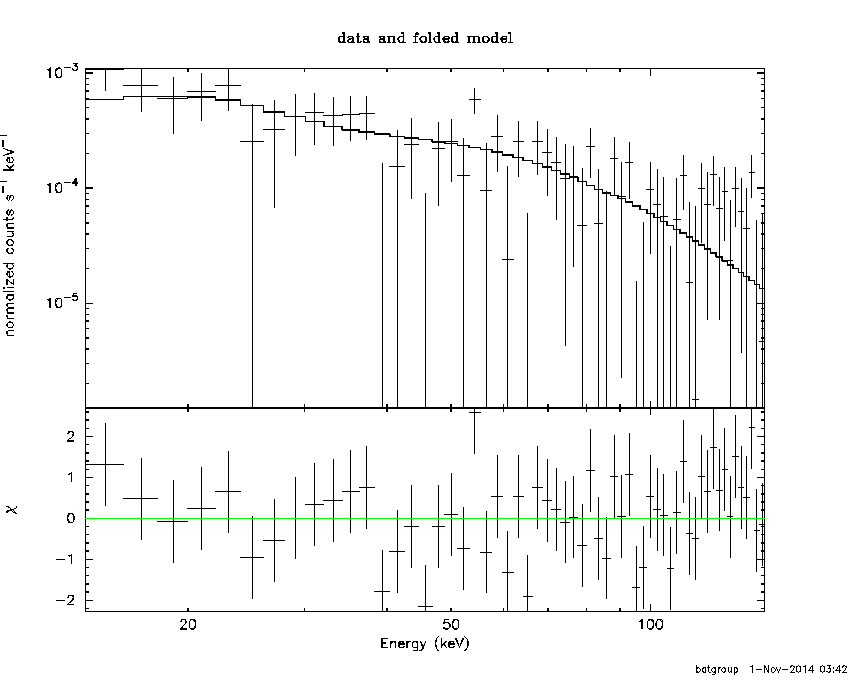```Time interval is from    1.208 sec. to   18.208 sec.

Spectral model in power-law:
------------------------------------------------------------
Parameters  : value       lower 90%   higher 90%
Photon index: 1.35719     ( -0.353972   0.350043 )
Norm@50keV  : 1.49855E-03    ( -0.000258466   0.000255211 )
------------------------------------------------------------
#Fit statistic : Chi-Squared =          56.28 using 59 PHA bins.
# Reduced chi-squared =         0.9874 for     57 degrees of freedom
# Null hypothesis probability =   5.020767e-01

Photon flux (15-150 keV)    in 17 sec: 0.180799 ( -0.0309972  0.0310266 ) ph/cm2/s
Energy fluence (15-150 keV)             : 2.48419e-07 ( -4.94542e-08  5.02777e-08 ) ergs/cm2

```
##### Cutoff power-law model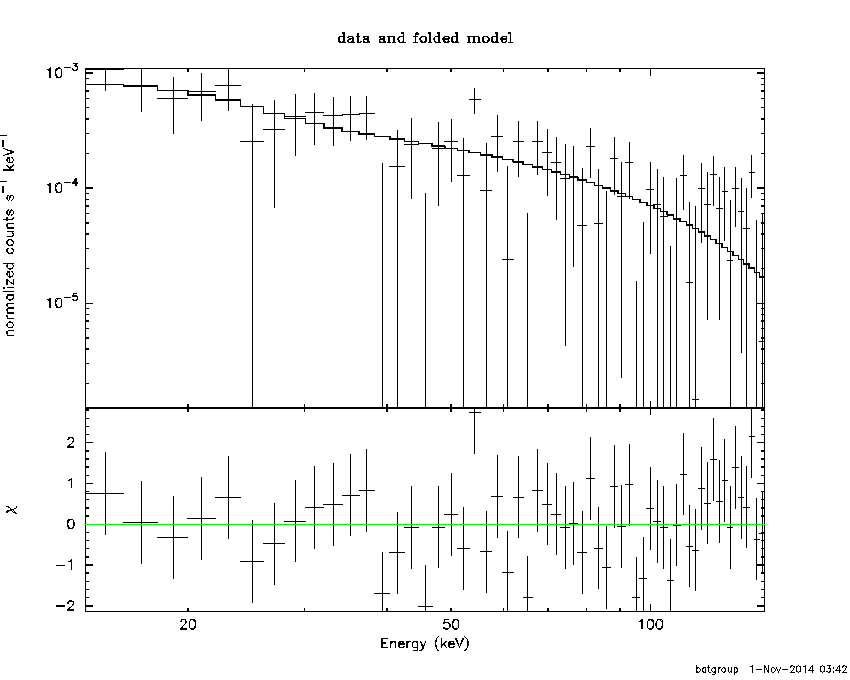```Time interval is from    1.208 sec. to   18.208 sec.
Spectral model in the cutoff power-law:
------------------------------------------------------------
Parameters  : value       lower 90%   higher 90%
Photon index: 2.46136     (     )
Epeak [keV] : 153.258      (   )
Norm@50keV  : 4.16428E-04    (     )
------------------------------------------------------------
#Fit statistic : Chi-Squared =          52.76 using 59 PHA bins.
# Reduced chi-squared =         0.9421 for     56 degrees of freedom
# Null hypothesis probability =   5.984269e-01

Photon flux (15-150 keV)    in 17 sec: 0.181359 ( -0.031333  0.030513 ) ph/cm2/s
Energy fluence (15-150 keV)             : 2.45925e-07 ( -4.79366e-08  4.74674e-08 ) ergs/cm2

```
##### Band function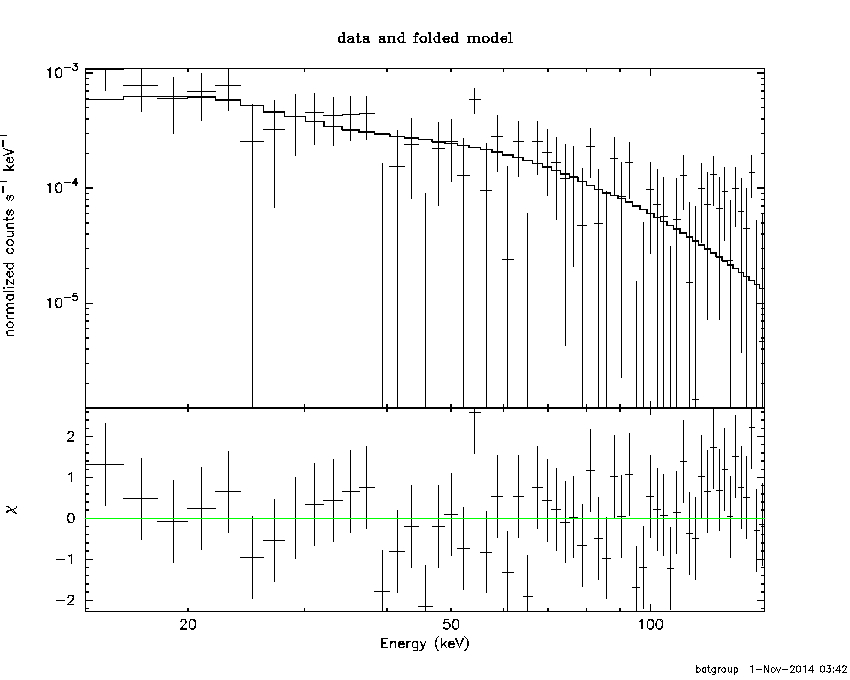```Time interval is from    1.208 sec. to   18.208 sec.

Spectral model in the Band function:
------------------------------------------------------------
Parameters  : value       lower 90%   higher 90%
alpha       : -1.09269   ( 1.09344   1.09344 )
beta        : -1.35666    ( 1.35701   0.353872 )
Epeak [keV] : 39.4123      ( -38.862 -38.862 )
Norm@50keV   : 2.87843E-03    ( -0.00163386   0.235484 )
------------------------------------------------------------
#Fit statistic : Chi-Squared =          56.28 using 59 PHA bins.
# Reduced chi-squared =          1.023 for     55 degrees of freedom
# Null hypothesis probability =   4.267852e-01

Photon flux (15-150 keV)    in 17 sec:  (    ) ph/cm2/s
Energy fluence (15-150 keV)             : 0 ( 0  0 ) ergs/cm2

```
##### Single BB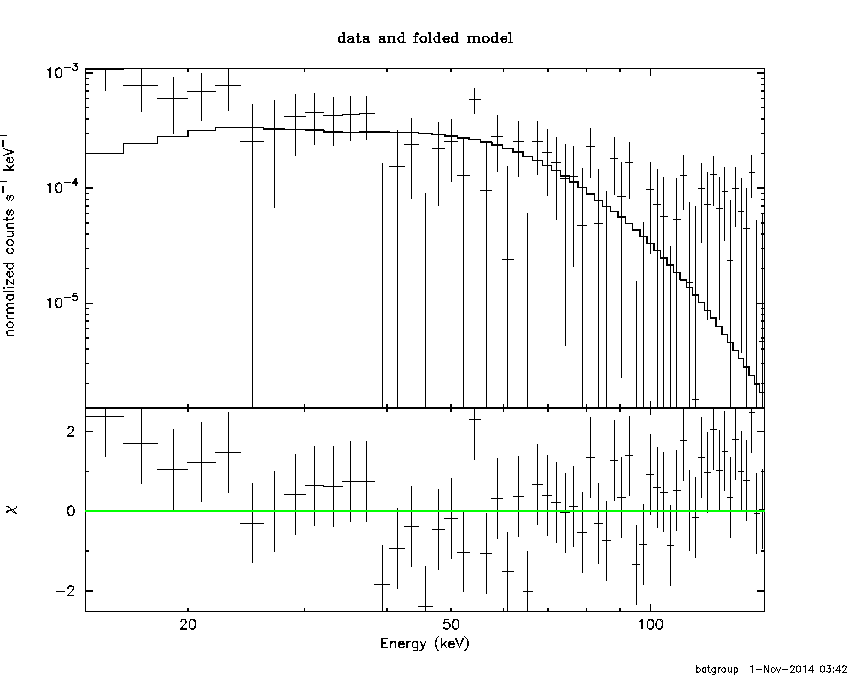```
Spectral model blackbody:
------------------------------------------------------------
Parameters  : value    Lower 90%  Upper 90%
kT [keV]    : 17.4517  (-5.3188  9.40188)
R^2/D10^2   : 1.15497E-02  (-0.0112679   0.0276054)
(R is the radius in km and D10 is the distance to the source in units of 10 kpc)
------------------------------------------------------------
#Fit statistic : Chi-Squared =          76.73 using 59 PHA bins.
# Reduced chi-squared =          1.346 for     57 degrees of freedom
# Null hypothesis probability =   4.178050e-02

Energy  Fluence     90% Error
[keV]   [erg/cm2]   [erg/cm2]
15- 25  1.209e-08   5.209e-09
25- 50  5.460e-08   1.969e-08
50-150  1.197e-07   4.422e-08
15-150  1.864e-07   5.500e-08
```
##### Thermal bremsstrahlung (OTTB)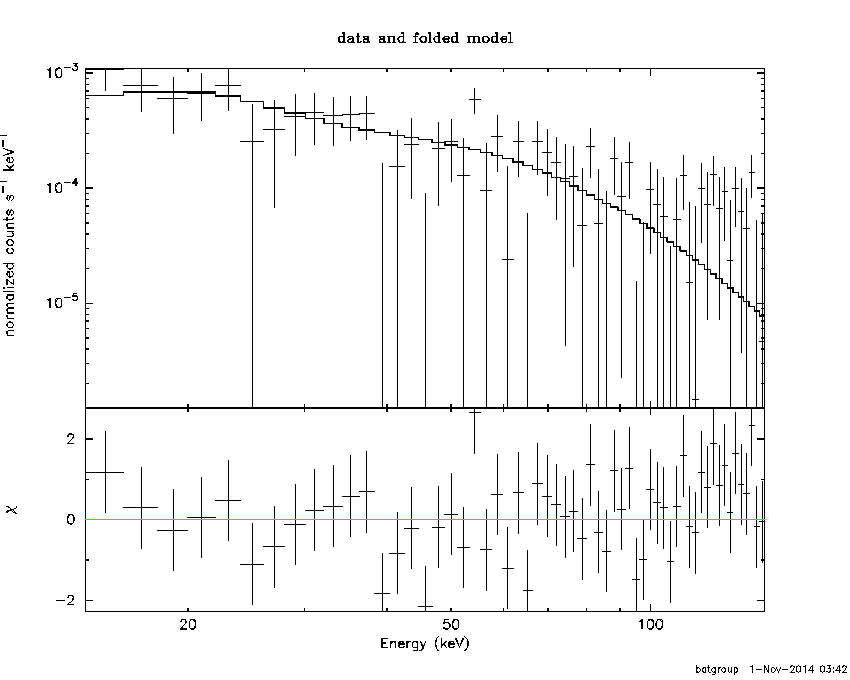```

Spectral model: thermal bremsstrahlung
------------------------------------------------------------
Parameters  : value         Lower 90%    Upper 90%
kT [keV]    : 200.000       (      )
Norm        : 0.736132       (-0.125273     0.125266)
------------------------------------------------------------
#Fit statistic : Chi-Squared =          59.28 using 59 PHA bins.
# Reduced chi-squared =          1.040 for     57 degrees of freedom
# Null hypothesis probability =   3.923450e-01

Energy  Fluence     90% Error
[keV]   [erg/cm2]   [erg/cm2]
15- 25  3.117e-08   1.819e-08
25- 50  5.996e-08   3.441e-08
50-150  1.315e-07   7.447e-08
15-150  2.226e-07   1.255e-07
```
##### Double BB```#Fit statistic : Chi-Squared =          53.76 using 59 PHA bins.
#Fit statistic : Chi-Squared =          53.07 using 59 PHA bins.
#Fit statistic : Chi-Squared =          52.99 using 59 PHA bins.
# Reduced chi-squared =         0.9994 for     55 degrees of freedom
# Reduced chi-squared =         0.9783 for     55 degrees of freedom
# Reduced chi-squared =         0.9774 for     55 degrees of freedom
# Reduced chi-squared =         0.9650 for     55 degrees of freedom
# Reduced chi-squared =         0.9634 for     55 degrees of freedom
# Null hypothesis probability =   4.759134e-01
# Null hypothesis probability =   5.203949e-01
# Null hypothesis probability =   5.222135e-01
# Null hypothesis probability =   5.485700e-01
# Null hypothesis probability =   5.519372e-01

Energy  Fluence     90% Error
[keV]   [erg/cm2]   [erg/cm2]
15- 25  3.303e-08   2.161e-08
25- 50  4.038e-08   2.267e-08
50-150  1.770e-07   1.103e-07
15-150  2.504e-07   1.429e-07
```

#### Peak spectrum fit

##### Power-law model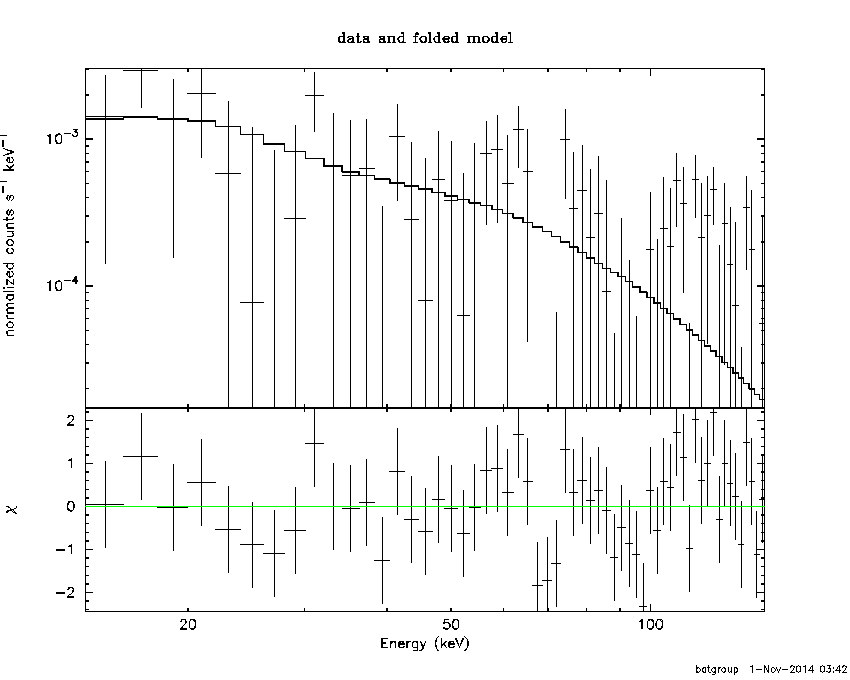```Time interval is from    4.208 sec. to    5.208 sec.

Spectral model in power-law:
------------------------------------------------------------
Parameters  : value       lower 90%   higher 90%
Photon index: 1.62821     ( -0.826163   0.942866 )
Norm@50keV  : 2.57680E-03    ( -0.00127754   0.00108823 )
------------------------------------------------------------
#Fit statistic : Chi-Squared =          56.27 using 59 PHA bins.
# Reduced chi-squared =         0.9872 for     57 degrees of freedom
# Null hypothesis probability =   5.024109e-01

Photon flux (15-150 keV)    in 1 sec: 0.334094 ( -0.126292  0.12622 ) ph/cm2/s
Energy fluence (15-150 keV)             : 2.40146e-08 ( -1.13501e-08  1.20207e-08 ) ergs/cm2

```
##### Cutoff power-law model```Time interval is from    4.208 sec. to    5.208 sec.
Spectral model in the cutoff power-law:
------------------------------------------------------------
Parameters  : value       lower 90%   higher 90%
Photon index: 3.97072     (     )
Epeak [keV] : 459.618      (   )
Norm@50keV  : 2.00166E-04    (     )
------------------------------------------------------------
#Fit statistic : Chi-Squared =          55.01 using 59 PHA bins.
# Reduced chi-squared =         0.9824 for     56 degrees of freedom
# Null hypothesis probability =   5.122034e-01

Photon flux (15-150 keV)    in 1 sec: 0.334200 ( -0.126101  0.126042 ) ph/cm2/s
Energy fluence (15-150 keV)             : 2.36351e-08 ( -1.09769e-08  1.19134e-08 ) ergs/cm2

```
##### Band function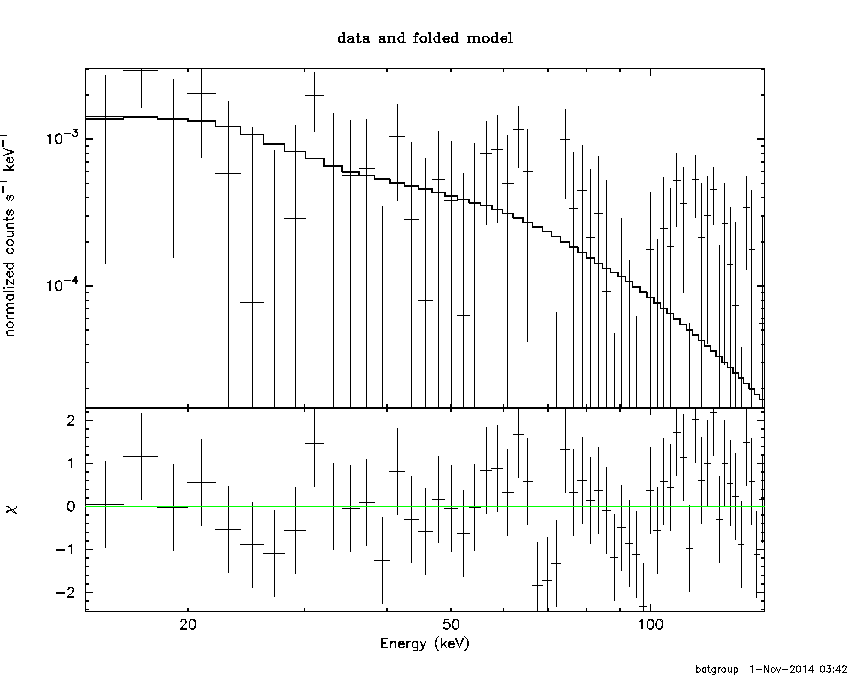```Time interval is from    4.208 sec. to    5.208 sec.

Spectral model in the Band function:
------------------------------------------------------------
Parameters  : value       lower 90%   higher 90%
alpha       : -1.31647   ( 1.31662   1.31662 )
beta        : -1.62778    ( 1.62866   0.825566 )
Epeak [keV] : 24.3629      ( -24.3838 -24.3838 )
Norm@50keV   : 5.62183E-03    ( -0.00562375   2356.03 )
------------------------------------------------------------
#Fit statistic : Chi-Squared =          56.27 using 59 PHA bins.
# Reduced chi-squared =          1.023 for     55 degrees of freedom
# Null hypothesis probability =   4.271119e-01

Photon flux (15-150 keV)    in 1 sec:  (    ) ph/cm2/s
Energy fluence (15-150 keV)             : 0 ( 0  0 ) ergs/cm2

```
##### Single BB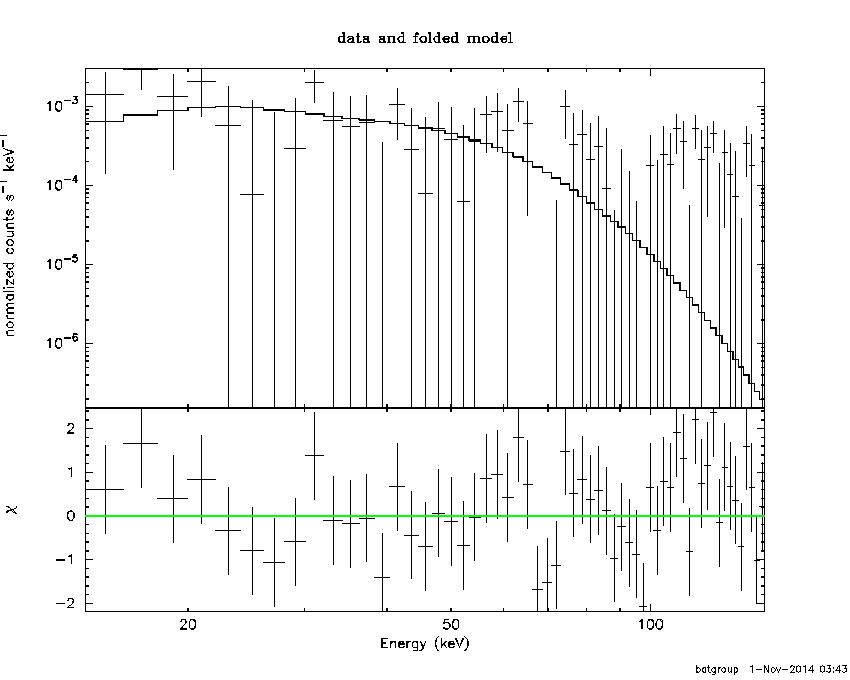```
Spectral model blackbody:
------------------------------------------------------------
Parameters  : value    Lower 90%  Upper 90%
kT [keV]    : 11.8492  (-5.62626  13.9349)
R^2/D10^2   : 7.96578E-02  (-0.0787687   0.747733)
(R is the radius in km and D10 is the distance to the source in units of 10 kpc)
------------------------------------------------------------
#Fit statistic : Chi-Squared =          60.15 using 59 PHA bins.
# Reduced chi-squared =          1.055 for     57 degrees of freedom
# Null hypothesis probability =   3.624529e-01

Energy  Fluence     90% Error
[keV]   [erg/cm2]   [erg/cm2]
15- 25  2.363e-09   1.564e-09
25- 50  7.326e-09   4.650e-09
50-150  6.132e-09   4.592e-09
15-150  1.582e-08   1.087e-08
```
##### Thermal bremsstrahlung (OTTB)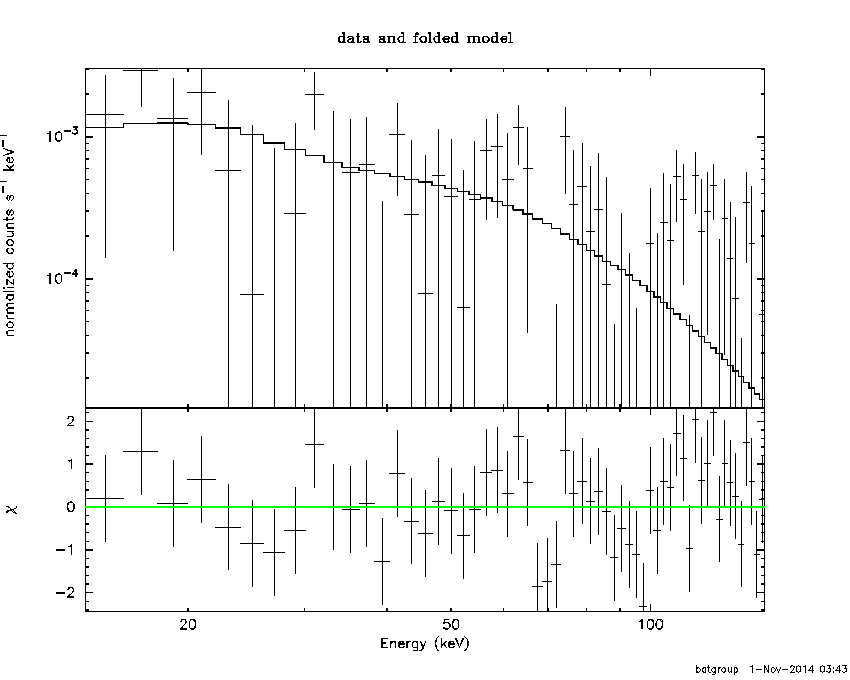```

Spectral model: thermal bremsstrahlung
------------------------------------------------------------
Parameters  : value         Lower 90%    Upper 90%
kT [keV]    : 199.362       (-199.363      -199.363)
Norm        : 1.33888       (-0.511825     1.07475)
------------------------------------------------------------
#Fit statistic : Chi-Squared =          56.79 using 59 PHA bins.
# Reduced chi-squared =         0.9964 for     57 degrees of freedom
# Null hypothesis probability =   4.828386e-01

Energy  Fluence     90% Error
[keV]   [erg/cm2]   [erg/cm2]
15- 25  3.334e-09   2.161e-09
25- 50  6.413e-09   4.024e-09
50-150  1.407e-08   9.355e-09
15-150  2.381e-08   1.527e-08
```
##### Double BB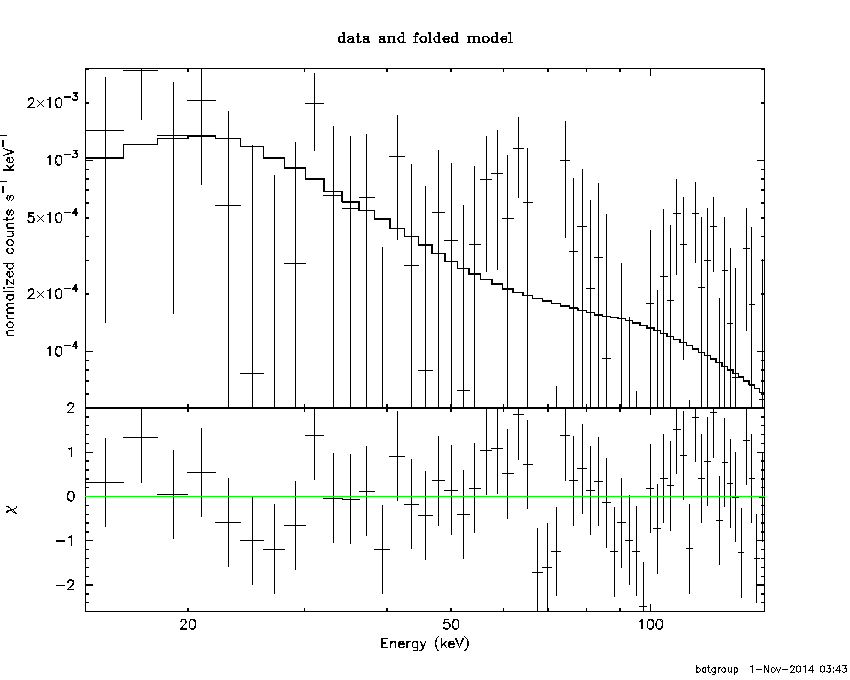```------------------------------------------------------------
#Fit statistic : Chi-Squared =          56.47 using 59 PHA bins.
#Fit statistic : Chi-Squared =          56.15 using 59 PHA bins.
#Fit statistic : Chi-Squared =          56.13 using 59 PHA bins.
#Fit statistic : Chi-Squared =          55.94 using 59 PHA bins.
# Reduced chi-squared =          1.027 for     55 degrees of freedom
# Reduced chi-squared =          1.021 for     55 degrees of freedom
# Reduced chi-squared =          1.021 for     55 degrees of freedom
# Reduced chi-squared =          1.017 for     55 degrees of freedom
# Null hypothesis probability =   4.197474e-01
# Null hypothesis probability =   4.316944e-01
# Null hypothesis probability =   4.323461e-01
# Null hypothesis probability =   4.392426e-01

Energy  Fluence     90% Error
[keV]   [erg/cm2]   [erg/cm2]
15- 25  4.249e-09   2.444e-09
25- 50  4.362e-09   2.616e-09
50-150  1.427e-08   9.855e-09
15-150  2.288e-08   1.319e-08
```
```Plot creation:
Sat Nov  1 03:43:40 EDT 2014
```